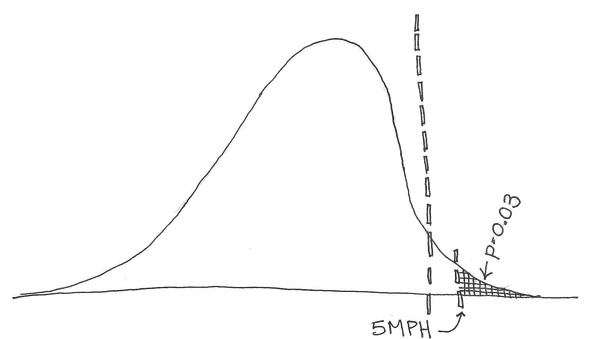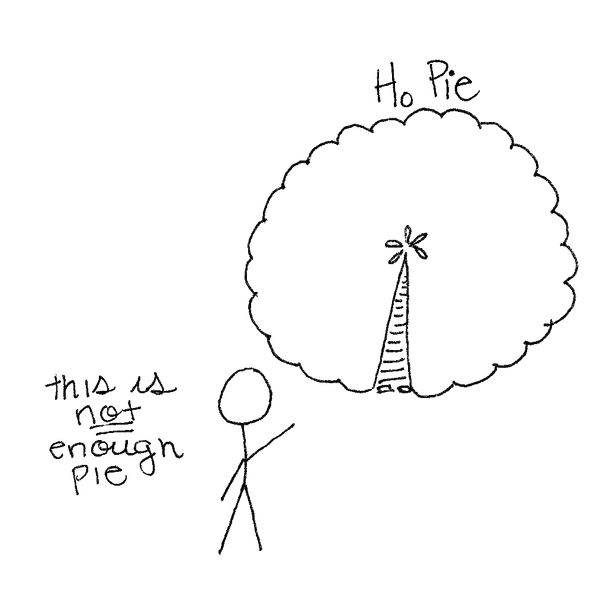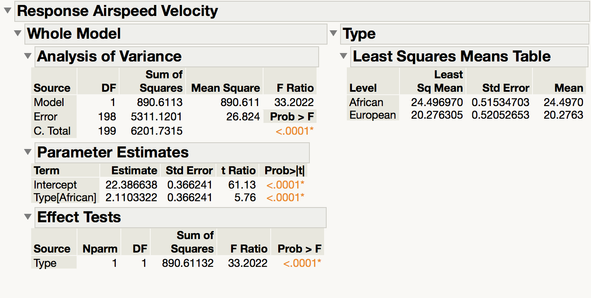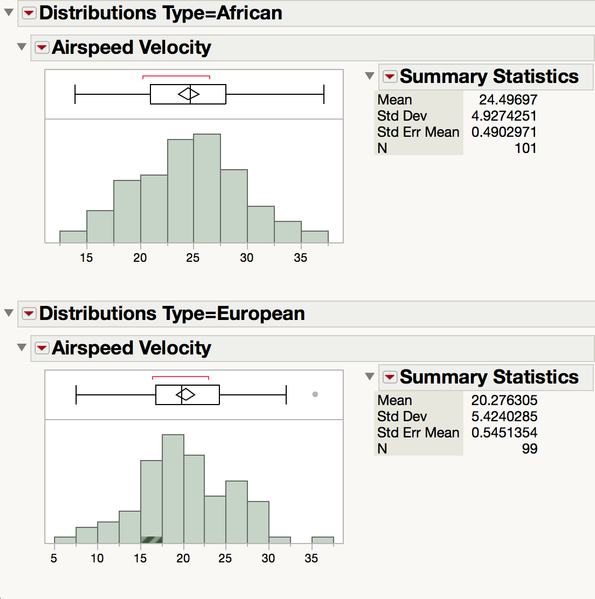Choose Language Hide Translation Bar
P-values

If you have ever taken any statistics or science classes, you’ve probably heard the term “p-value.” Technically, the p means probability: A p-value is the probability of something happening, but I want to tell you that p can stand for a lot of things like Probability, Perplexing, and Part.

### Probability

Let’s start with the technical stuff. When we do a hypothesis test, the first thing we do is assume that the null hypothesis is true.Then we collect some data. I’m interested in whether there is a difference between the airspeed velocity of African vs. European swallows. I grab my bird-catching kit and get a bunch of birds, measure their air speed velocity, and find that on average African swallows are 5 mph faster than their European counterparts.Then I ask “if there were no difference between African and European swallows and I just grab some birds at random, what percent of the time am I going to get two groups of swallows with an average difference greater than 5 mph?” The probability of that happening is my p-value: the probability of getting a value as extreme or more extreme than my data — assuming that the null hypothesis is true.But how do we know how likely all these things are? For each test statistic, we have a distribution. To name a few, we have a z-distribution, t-distribution, F-distribution, and Chi squared-distribution. All of them have different shapes — some even change depending on how big our sample size is — but they all tell us the relative probability of getting all the different values for each test statistic.So we calculate a test statistic — say a t-value — based on our data and then mentally draw a line at that value. Our p-value is the proportion of the distribution that is more extreme than that line.It’s like a pie chart — or better yet a pie. Your p-value is the proportion of the null hypothesis pie you get in your slice. As your slice of pie gets smaller and smaller, you get more and more upset until it is so small that finally you say “screw this pie, I’ll go get some alternative hypothesis pie!”### Perplexing

Maybe it seems simple, but there has been a lot of controversy over the p-value lately. The problem stems from a widespread misunderstanding of the p-value. Here is a short list of things a p-value is not:

• The probability that the null hypothesis is true.
• The probability that your results are false if you get a significant p-value.
• The probability that there actually is an effect if you get an insignificant p-value.
• An indication of how practically significant your results are.

So why are p-values so perplexing? One reason is that they are not very intuitive. We want statistics to tell us the chance that our hypothesis is correct — or conversely the chance that the null hypothesis is incorrect — but that’s not what frequentist hypothesis tests tell you. In probability notation, this is:However, frequentist tests tell us the probability of getting our data, given that the null hypothesis is true:You can see that these two are not the same thing, but we treat P(data | H0) as a proxy for P(H0 | data), which contributes both to the confusion and to misstatements about what a significant p-value means. If p-values aren’t taught clearly, it get’s confusing, but there's a difference between the probability of being a child given that you’re at Chuck-E-Cheese, and the probability that you’re at Chuck-E-Cheese given that you’re a child.

### Part

So what do we do? Do we go the way of some academic journals and scrap p-values? I argue no. The final p is part. P-values are only part of the picture that we need in order to understand our data and what they say about the world around us; they tell us how likely it is that something just happened by chance.

Another important part is effect size. Effect sizes measure how big your effect is relative to how much things vary in the population. If I found that blondes are heavier on average by 3 pounds, that would be more interesting if I were talking about newborns than all adults, because newborns vary less in weight than adults. Effect sizes take your effect (in our case, 3 pounds) and divide it by how variable your population is (a lot for adults, a little for newborns).

Together, p-values and effect sizes can give you a better picture of your data and the world around you. They tell you more about practical significance. Saying that you found a 3-pound statistically significant difference in weight doesn’t mean much until you know whether that’s for babies (who vary very little) or adults (who vary a lot).

So before you use or talk about p-values, just remember that they're the probability of getting your data if the null hypothesis is true, a sometimes perplexing thing to talk about, and only part of the big picture.

But back to those swallows (I swear no birds were harmed in the writing of this article)...When you're looking at an ANOVA on Airspeed Velocity and see a significant effect of swallow type, you can use the Analyze>Distribution platform in JMP or the Calculate Effect Sizes Add-In to look at the effect sizes of your data as well. (JMP file attached; #AfricanSwallowsForAllCoconutTransport.)Note: A version of this article was originally posted with our friends Partially Derivative.

Article Labels

There are no labels assigned to this post.

Level III

Thanks for the explaination!!Staff

Thanks very much Chelsea for the nice article and love the illustrations!  As controversies around p-values continue to swirl, we want to make sure everyone knows how to properly interpret them in the many places they appear across JMP platforms.

A note of clarification regarding frequentist versus Bayesian approaches and the notation P(data|H0) versus P(H0|data): Probability theory is mathematical and can be applied in many different ways to real-life problems.  A Bayesian method typically defines a probability measure P() as a subjective degree of belief, expressing uncertainty about propositions in a statistical model over both data and parameters.   All of the rigorous math and a beautifully coherent theory has been developed by such greats as Laplace and de Finetti, typically motivated by betting scenarios, and computable with Markov Chain Monte Carlo techniques.   Two big gotchas with Bayesian methods are the need to specify priors and this tacitly subtle but very important way of expressing P() epistemically.   With all this in place, you nicely describe the danger of transposing the conditional, and to be sure to understand what is on the left and right of the bar in Bayesian P(|) notation.

In contrast, frequentist methods map to the real world by setting up a different probability measure, one that captures frequency variation in the presumed data generating mechanism of scientific systems we study.   Imagine all of the amazingly tiny forces that accumulate when measuring swallow airspeed velocity: wind speed via air molecules, micro muscle fibers, instrument tech, and we can even push all these further to the quantum level.   Here the central limit theorem and laws of large numbers literally come to life and can provide reasonable justification for a normal theory linear model with P() defined according to real-world frequencies.   With this probability measure, unknown parameters in the model map to physical constants of a sort, and as such, P(H0) and P(H0|data) (prior and posterior) really don't make sense.  The convention in the statistics literature is to use either a semicolon or subscript notation when assuming H0 is true, and to use phrases like "under H0".   Frequentist methods like the p-value, pioneered by Fisher, Neyman, and Pearson, focus on controlling error rates and involve related concepts like confidence intervals, power, and severity (see Deborah Mayo's book and blog).

I find the distinction above helpful while wrestling with commentaries on p-values, and of course we can try to make connections and reconciliations between Bayesian and frequentist probabilities once appreciating their differences.  I'd also like to caution all readers to be wary of any statement that refers to the "the probability".  The author typically has either a Bayesian or frequentist probability measure in mind and this is often not stated, and sometimes they even freely flow back and forth without warning.  Our shining example here is a p-value, which is a frequentist statistic, but can often be interpreted Bayesianly if desired.